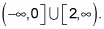##### Linear Algebra For Dummies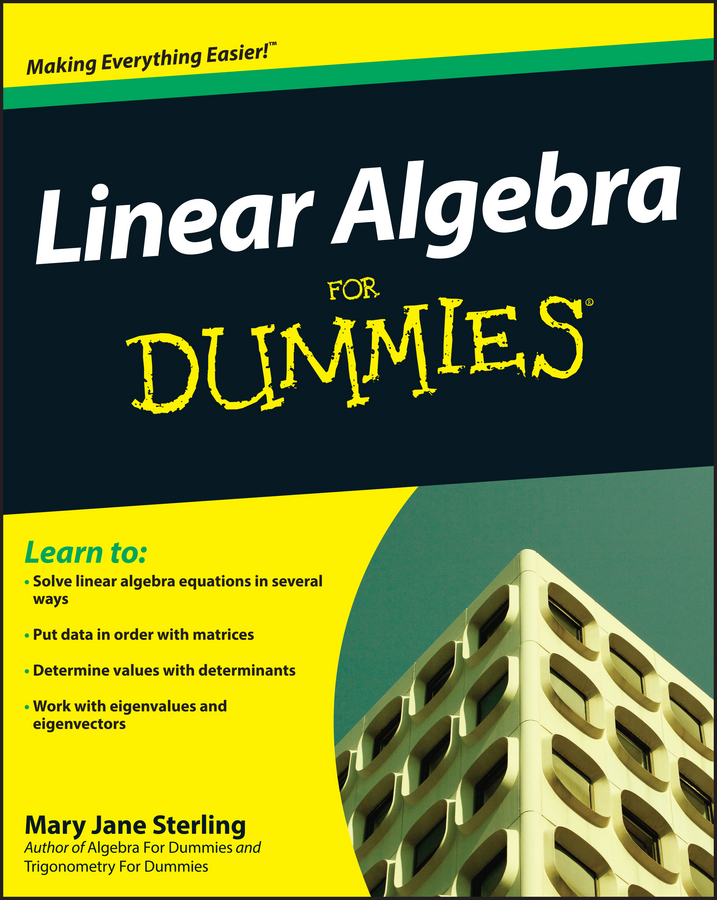If you have to change the amplitude, period, and position of a secant or cosecant graph, your best bet is to graph their reciprocal functions and transform them first. The reciprocal functions, sine and cosine, are easier to graph because they don't have as many complex parts (no asymptotes, basically). If you can graph the reciprocals first, you can deal with the more complicated pieces of the secant/cosecant graphs last.

For example, take a look at the graph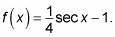1. Graph the transformed reciprocal function y = 1/4 cos x – 1.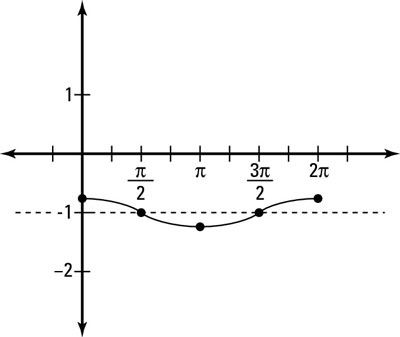Look at the reciprocal function for secant, which is cosine. Pretend just for a bit that you're graphing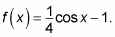Follow all the rules for the cosine graph in order to end up with a graph that looks like the one in the figure.

2. Sketch the asymptotes of the transformed reciprocal function.

Wherever the transformed graph involving cosine crosses its sinusoidal axis, you have an asymptote in the graph involving secant. You see that the cosine graph crosses the sinusoidal axis when x = pi/2 and 3pi/2.

3. Find out what the graph looks like between each asymptote.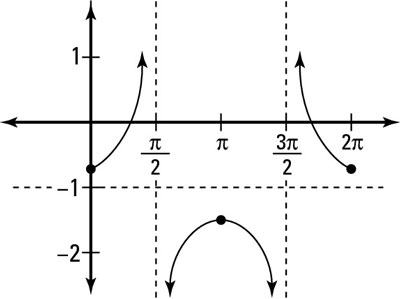Now that you've identified the asymptotes, you simply figure out what happens on the intervals between them. The finished graph,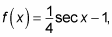ends up looking like the one in the figure.

4. State the domain and range of the transformed function.

Because the new transformed function may have different asymptotes than the parent function for secant and it may be shifted up or down, you may be required to state the new domain and range.

This example,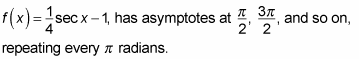Therefore, the domain is restricted to not include these values and is written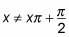where x is an integer. In addition, the range of this function changes because the transformed function is shorter than the parent function and has been shifted down 2. The range has two separate intervals,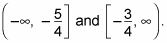You can graph a transformation of the cosecant graph by using the same steps you use when graphing the secant function, only this time you use the sine function to guide you.

The shape of the transformed cosecant graph should be very similar to the secant graph, except the asymptotes are in different places. For this reason, be sure you're graphing with the help of the sine graph (to transform the cosecant graph) and the cosine function (to guide you for the secant graph).

For example, graph the transformed cosecant graph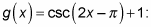1. Graph the transformed reciprocal function.

Look first at the function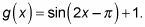The rules to transforming a sine function tell you to first factor out the 2 and get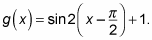It has a horizontal shrink of 2, a horizontal shift of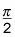to the right, and a vertical shift of up 1. The figure shows the transformed sine graph.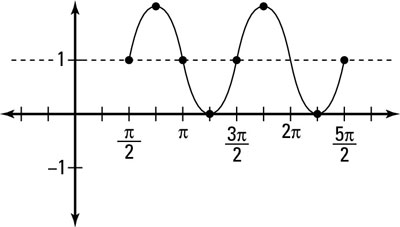2. Sketch the asymptotes of the reciprocal function.

The sinusoidal axis that runs through the middle of the sine function is the line y = 1. Therefore, an asymptote of the cosecant graph exists everywhere the transformed sine function crosses this line. The asymptotes of the graph involving cosecant are at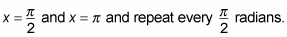3. Figure out what happens to the graph between each asymptote.

You can use the transformed graph of the sine function to determine where the cosecant graph is positive and negative. Because the graph of the transformed sine function is positive in between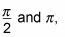the cosecant graph is positive as well and extends up when getting closer to the asymptotes. Similarly, because the graph of the transformed sine function is negative in between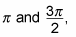the cosecant is also negative in this interval. The graph alternates between positive and negative in equal intervals forever in both directions.

The figure shows the transformed cosecant graph.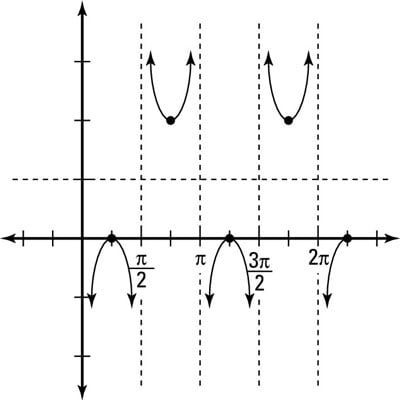4. State the new domain and range.

Just as with the transformed graph of the secant function, you may be asked to state the new domain and range for the cosecant function. The domain of the transformed cosecant function is all values of x except for the values that are asymptotes. From the graph, you can see that the domain is all values of x, where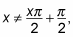where x is an integer. The range of the transformed cosecant function is also split up into two intervals: